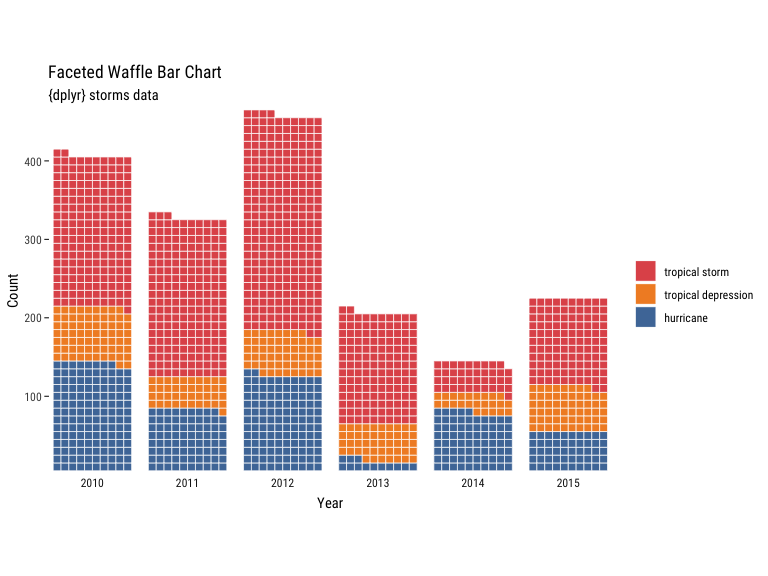# Building Waffle Charts

library(hrbrthemes)
library(waffle)
library(ggplot2)
library(dplyr)

### Our example data

three_states <- sample(state.name, 3)

data.frame(
states = factor(rep(three_states, 3), levels = three_states),
vals = c(10, 20, 30, 6, 14, 40, 30, 20, 10),
col = rep(c("blue", "black", "red"), 3),
fct = c(rep("Thing 1", 3), rep("Thing 2", 3), rep("Thing 3", 3))
) -> xdf

xdf
##     states vals   col     fct
## 1 Nebraska   10  blue Thing 1
## 2     Ohio   20 black Thing 1
## 3  Wyoming   30   red Thing 1
## 4 Nebraska    6  blue Thing 2
## 5     Ohio   14 black Thing 2
## 6  Wyoming   40   red Thing 2
## 7 Nebraska   30  blue Thing 3
## 8     Ohio   20 black Thing 3
## 9  Wyoming   10   red Thing 3

### Single waffle setup

We’ll use this as a base for some of the examples to enable focusing on tweaking the contents of geom_waffle():

xdf %>%
count(states, wt = vals) %>%
ggplot(aes(fill = states, values = n)) +
expand_limits(x=c(0,0), y=c(0,0)) +
coord_equal() +
labs(fill = NULL, colour = NULL) +
theme_ipsum_rc(grid="") +
theme_enhance_waffle() -> waf

### Plain waffles

waf +
geom_waffle(
n_rows = 20, size = 0.33, colour = "white", flip = TRUE
)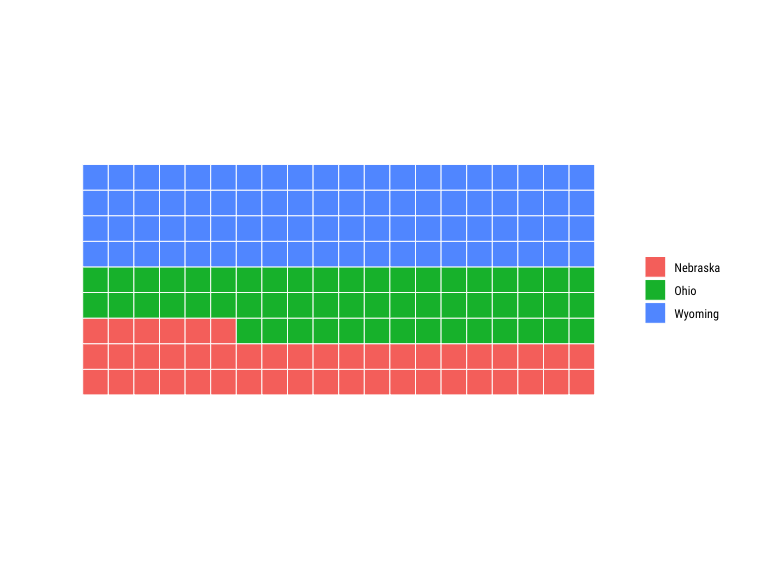### Proportional waffles

This likely should be the default. Waffles work best when they are square (makes it easier to compare parts to whole which is the purpose of the chart). You could do this normalization prior to passing data into geom_waffle() or let it do it for you with the make_proportional parameter.

waf +
geom_waffle(
n_rows = 10, size = 0.33, colour = "white", flip = TRUE,
make_proportional = TRUE
)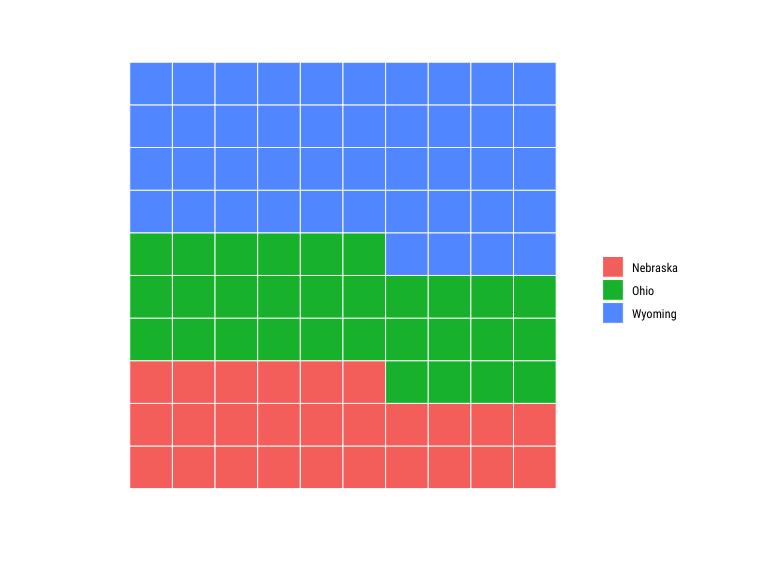### Thicker lines

Need to be careful as this can shift perception to the background grid vs the data encoding you’re trying to present.

waf +
geom_waffle(
n_rows = 10, size = 3, colour = "white",
make_proportional = TRUE
)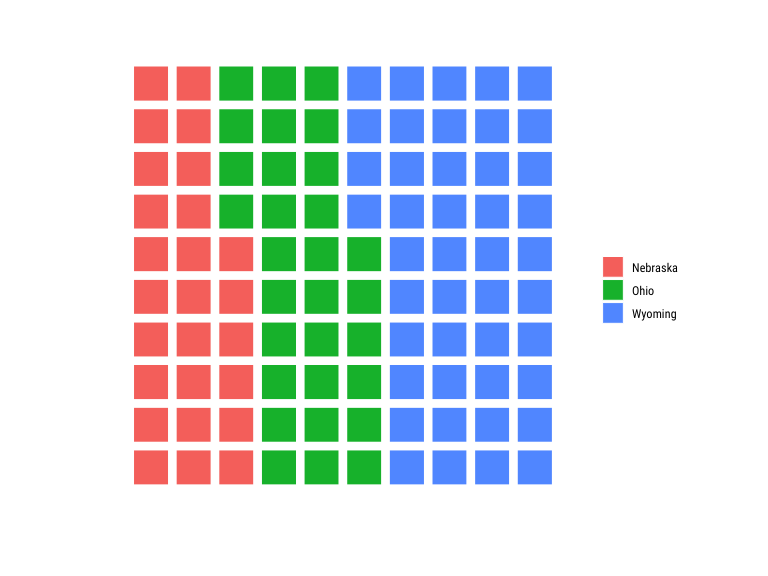### Changing the line color

You can use this to match any background you’re going to use or to just provide a different aesthetic feel. Note that the same problem can occur here as in the “bigger lines” case and attention can be inadvertedly shifted to the grid lines vs the colored proportions.

waf +
geom_waffle(
n_rows = 10, size = 0.33, colour = "black",
make_proportional = TRUE
)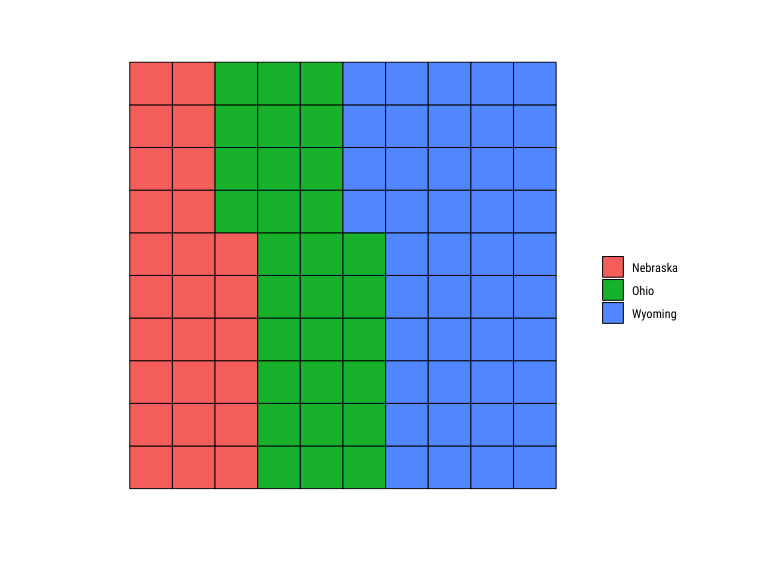We can also “fill in the lines” but that pretty much makes it not a waffle chart:

waf +
geom_waffle(
aes(colour = states),
n_rows = 10, size = 0.33, make_proportional = TRUE
)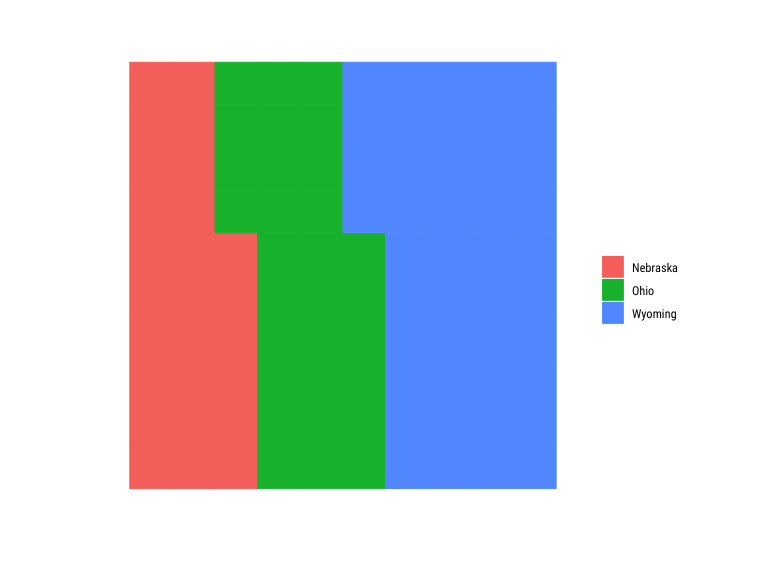You can also map the colour aesthetic to a column which can help make a “highlight” effect, but that’s going to also contribute to perception skew:

waf +
geom_waffle(
aes(colour = states),
n_rows = 10, size = 0.45, make_proportional = TRUE
) +
scale_colour_manual(
values = c(alpha("black", 1/3), "black", alpha("black", 1/3))
)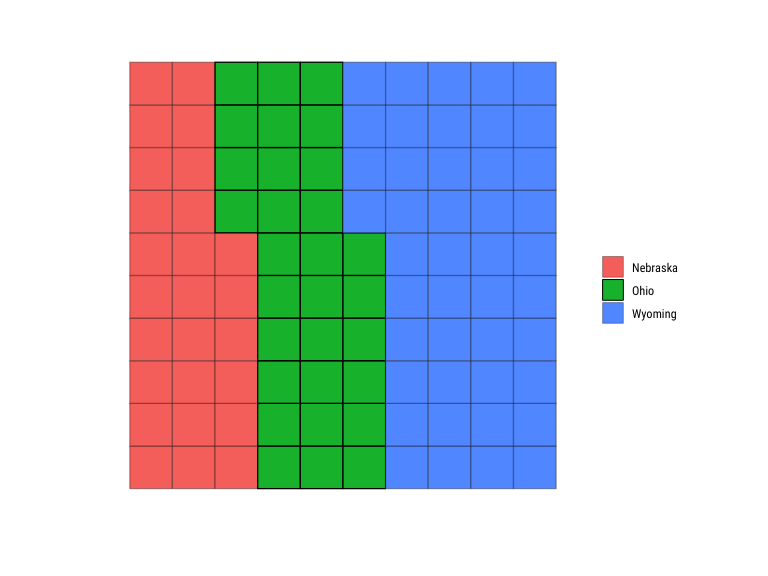You can possibly correct for perception skew by shrinking the width and the height of each cell to make some room for the strokes:

waf +
geom_waffle(
aes(colour = states),
n_rows = 10, size = 0.3, make_proportional = TRUE,
height = 0.9, width = 0.9
) +
scale_colour_manual(
values = c("white", "black", "white")
)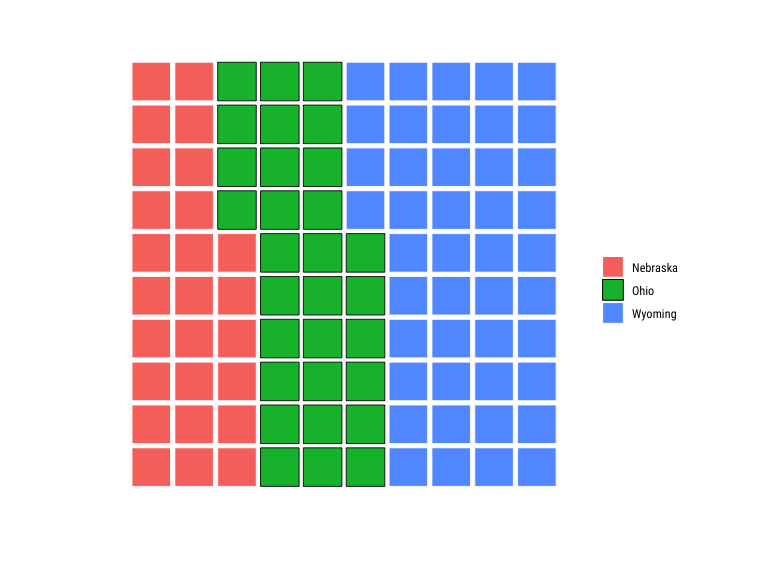You might be better off just changing the alpha value’s though:

waf +
geom_waffle(
n_rows = 10, size = 0.3, make_proportional = TRUE,
height = 0.9, width = 0.9
) +
scale_fill_manual(
values = c(alpha("#f8766d", 1/3), "#00ba38", alpha("#619cff", 1/3))
)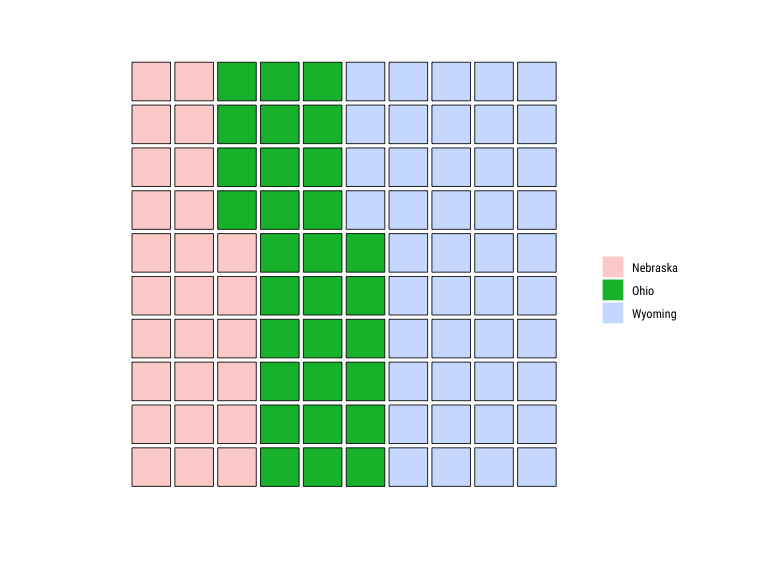### Hip to not be square?

To mix things up you can also round out the corners by specifying a grid::unit() value to the radius parameter. This isn’t generally recommended as the goal is to enable quick mental perception for parts to whole and the rounded corners can delay and/or skew said interpretation.

Here that is with and without proportional waffles:

waf +
geom_waffle(
n_rows = 10, size = 0.5, colour = "white",
make_proportional = TRUE,
)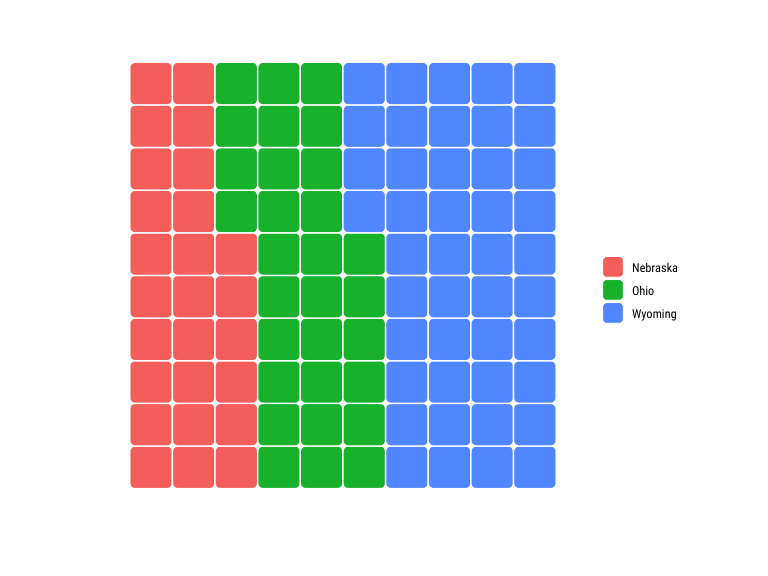waf +
geom_waffle(
n_rows = 10, size = 0.5, colour = "white",
)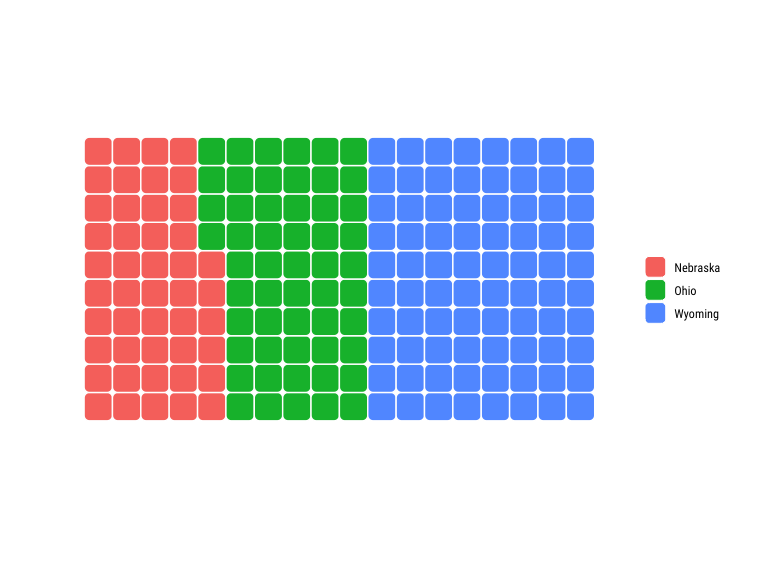Also, think twice when changing the stroke color as it continues to contribute to perception skew. Consider shrinking the cells to add more space between them if you choose to do this:

waf +
geom_waffle(
n_rows = 10, size = 1, colour = "black",
make_proportional = TRUE,
height = 0.8, width = 0.8
)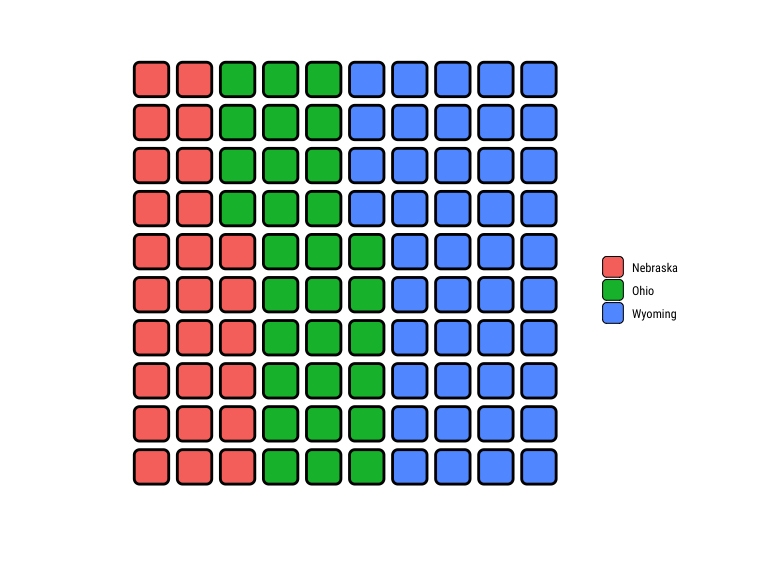You can also use this for the same highlight effect as above:

waf +
geom_waffle(
aes(colour = states),
n_rows = 10, size = 0.4, make_proportional = TRUE,
height = 0.9, width = 0.9
) +
scale_colour_manual(
values = c("black", "white", "white")
)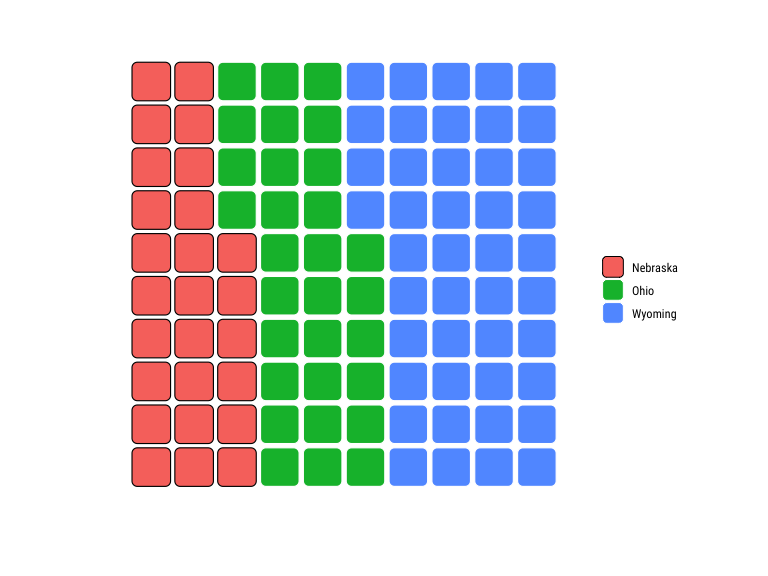waf +
geom_waffle(
n_rows = 10, size = 1, color = "white", make_proportional = TRUE,
height = 1, width = 1
) +
scale_fill_manual(
values = c("#f8766d", alpha("#00ba38", 1/3), alpha("#619cff", 1/3))
)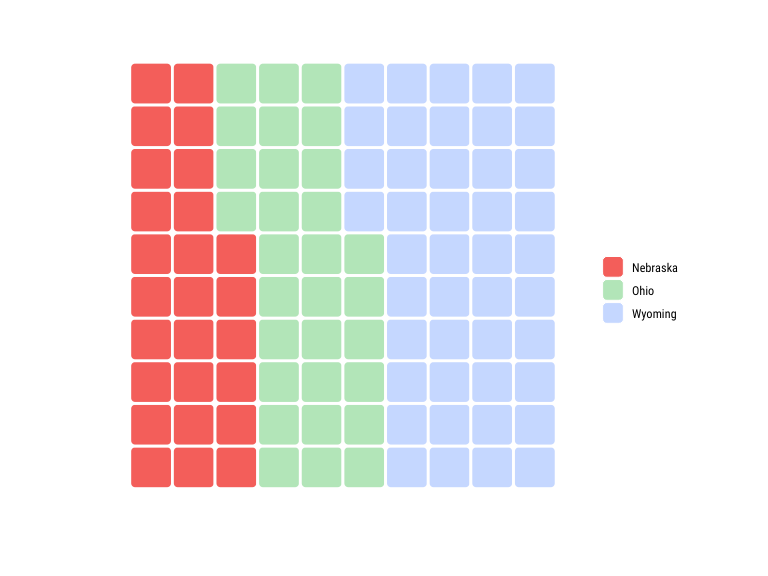### Basic waffle bar chart

You can make a bar-like chart with the waffles by using facet wrapping and hacking on strip spacing:

waf +
geom_waffle(
n_rows = 5, color = "white", show.legend = FALSE, flip = TRUE
) +
facet_wrap(~states) +
theme(panel.spacing.x = unit(0, "npc")) +
theme(strip.text.x = element_text(hjust = 0.5))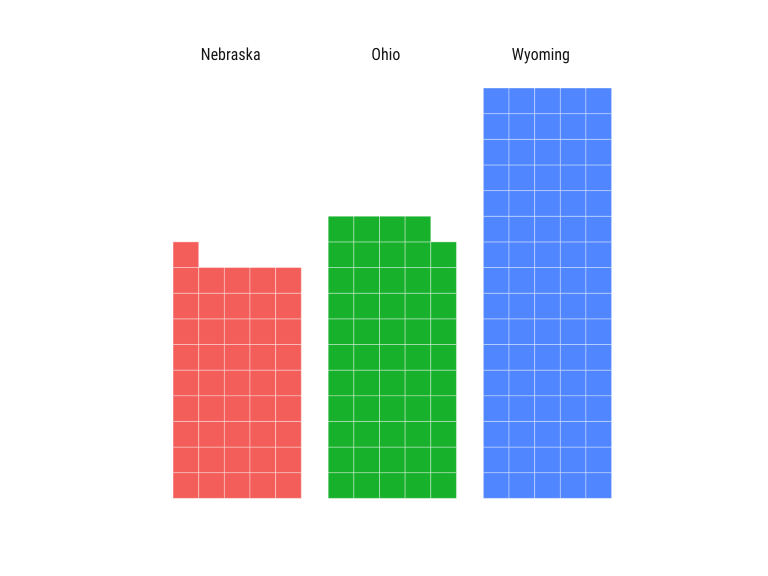### Waffle buffet setup

Since you now know we can use faceting, we can go all sorts of crazy. We’ll do another setup for this waffle buffet:

xdf %>%
ggplot(aes(fill = states, values = vals)) +
expand_limits(x=c(0,0), y=c(0,0)) +
coord_equal() +
labs(fill = NULL, colour = NULL) +
theme_ipsum_rc(grid="") +
theme_enhance_waffle() -> buf

### Faceting using another variable

If you have parts-of-a-whole groups you want to compare across observations you can facet on another variable

buf +
geom_waffle(
color = "white", size = 0.33
) +
facet_wrap(~fct) +
theme(strip.text.x = element_text(hjust = 0.5))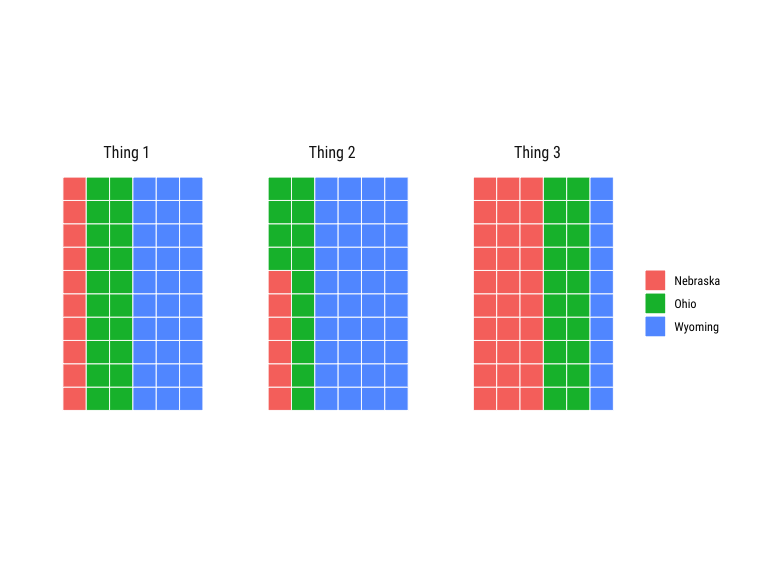Again, waffles generally work better when they are square and each one sums to 100 and this is even more true in a buffet grid of waffles:

buf +
geom_waffle(
color = "white", size = 0.33,
make_proportional = TRUE, n_rows = 10
) +
facet_wrap(~fct) +
theme(legend.position = "bottom") +
theme(strip.text.x = element_text(hjust = 0.5))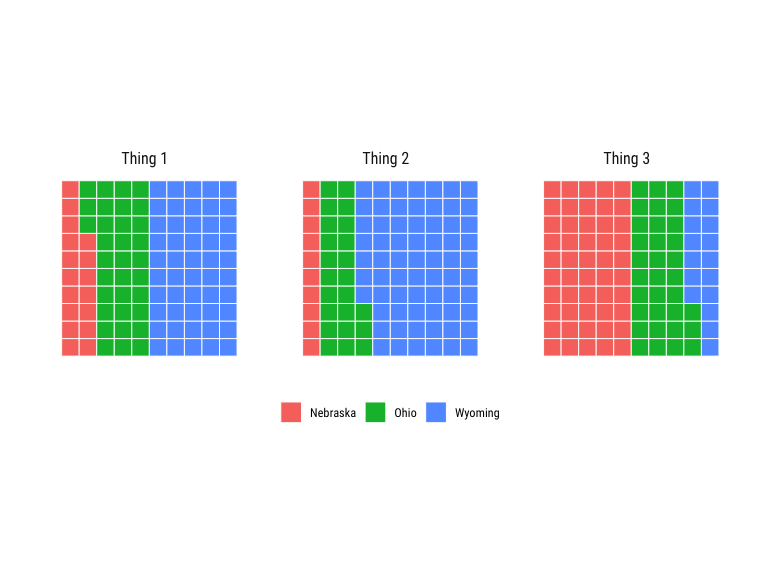They can be rounded tiles as well:

buf +
geom_waffle(
color = "white", size = 0.33,
make_proportional = TRUE, n_rows = 10,
) +
facet_wrap(~fct) +
theme(legend.position = "bottom") +
theme(strip.text.x = element_text(hjust = 0.5))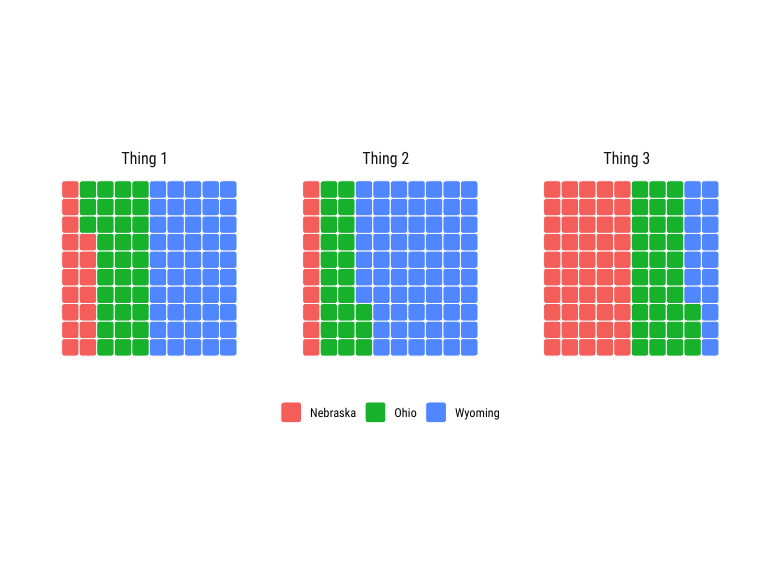And, you can do the highlight hack:

buf +
geom_waffle(
color = "white", size = 0.33,
make_proportional = TRUE, n_rows = 10,
) +
facet_wrap(~fct) +
scale_fill_manual(
values = c("#f8766d", alpha("#00ba38", 1/3), alpha("#619cff", 1/3))
) +
theme(legend.position = "bottom") +
theme(strip.text.x = element_text(hjust = 0.5))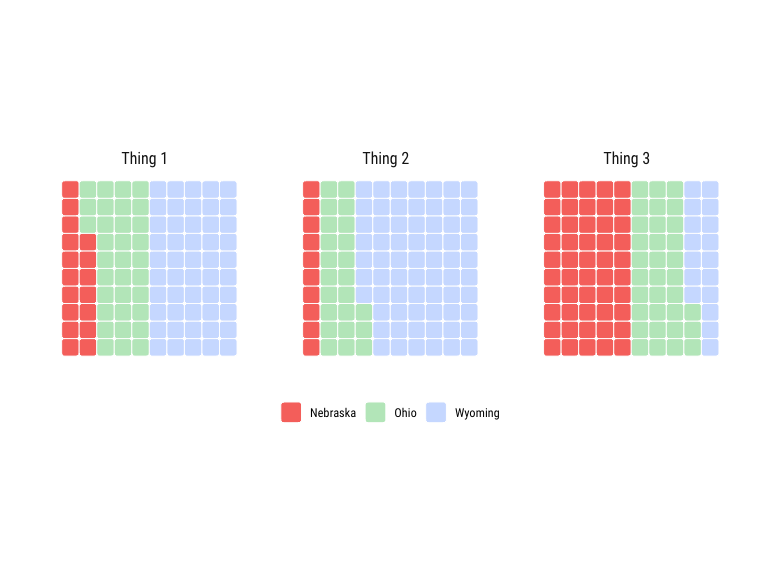If you aren’t going to use proportional waffle buffet charts consider altering the aesthetics to make them waffle bars instead:

buf +
geom_waffle(
color = "white", size = 0.33, n_rows = 4, flip = TRUE
) +
facet_wrap(~fct) +
theme(legend.position = "bottom") +
theme(strip.text.x = element_text(hjust = 0.5))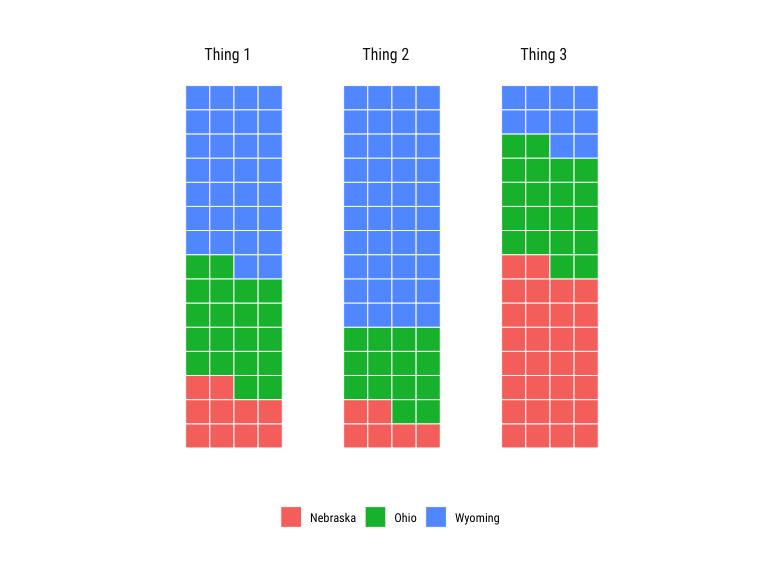### Over the top

storms %>%
filter(year >= 2010) %>%
count(year, status) -> storms_df

ggplot(storms_df, aes(fill = status, values = n)) +
geom_waffle(color = "white", size = .25, n_rows = 10, flip = TRUE) +
facet_wrap(~year, nrow = 1, strip.position = "bottom") +
scale_x_discrete() +
scale_y_continuous(labels = function(x) x * 10, # make this multiplyer the same as n_rows
expand = c(0,0)) +
ggthemes::scale_fill_tableau(name=NULL) +
coord_equal() +
labs(
title = "Faceted Waffle Bar Chart",
subtitle = "{dplyr} storms data",
x = "Year",
y = "Count"
) +
theme_minimal(base_family = "Roboto Condensed") +
theme(panel.grid = element_blank(), axis.ticks.y = element_line()) +
guides(fill = guide_legend(reverse = TRUE))